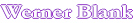Home viscal10                 Viscosity of a blend of two polymer solutions This program calculates the viscosity of a blend of two polymer solutions at different non-volatile content and temperature. As input two viscosity and solids measurement of each polymer are required. A combination of the William, Landel and Ferry (WLF) equation and the Fox equation is used in the calculation. The constants in the WLF equation are according to Nielsen and reasonable accurate for most polymers. For a specific polymer the constants can be adjusted to increase the accuracy. The assumption is made that the Tg of a polymer solution decreases linear with the solvent fraction. Tgs = Co +C1Ws, Tgs = solution Tg, Co, C1= constants for polymer solution, Ws = weight fraction of solvent. 1/Tgb = W1/Tg1 + W2/Tg2 , Tgb = Tg of polymer solution blend, W1,2 = weight fraction of polymer solution 1, 2, Tg1,2 Tg of polymer solution 1 an 2. Measured data Solids, % Visc., cps Temp., şC. Solvent, % Poise Visc. log T-Tg Tg Polymer A sol.1 Polymer A sol.2 Polymer B sol.1 Polymer B sol.2 Constants Polymer 1 Co = WLF Log η= C-A(T-Tg)/(B+(T-Tg)) C1 = equation C= Universal constants according to Nielsen Polymer 2 Co = A= C1 = B= Results Enter desired blend Poly B weight fraction: Polymer A Constants Co Polymer B of blend C1 Solids % Viscosity, Poise log Temp. şC: Viscosity, cps
 M.L.Williams, R.F.Landel and  J.D.Ferry, J.Am.Chem.Soc.77,3701(1955) L.E.Nielsen, Polymer Rheology, Marcel Dekker, 1977,pp.33,74,133

Automatic recalculation

 Last edited on: October 20, 2010 Copyright®, Design, Layout and Technical Content by: